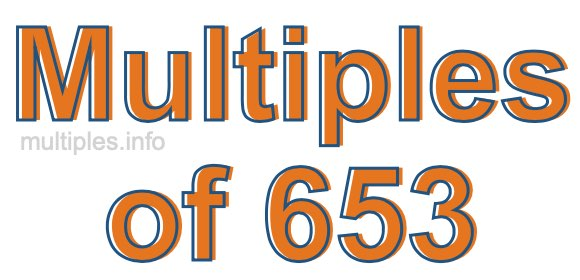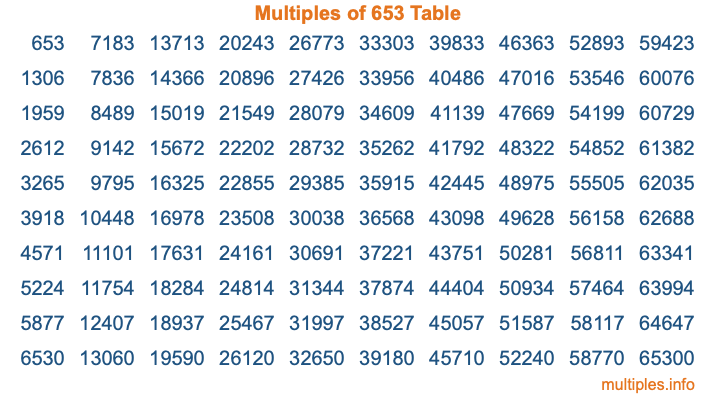Multiples of 653Welcome to the Multiples of 653 page. Here we will first teach you everything you will ever need to know about the multiples of 653, and then give you a study guide summary of everything we taught you to make sure you remember it all. Use this page to look up facts and learn information about the multiples of 653. This page will make you a multiples of six hundred fifty-three expert!

Definition of Multiples of 653
Multiples of 653 are all the numbers that when divided by 653 equal an integer. Each of the multiples of 653 are called a multiple. A multiple of 653 is created by multiplying 653 by an integer.

Therefore, to create a list of multiples of 653, you start with 1 multiplied by 653, then 2 multiplied by 653, then 3 multiplied by 653, and so on for as long as you want. Thus, the list of the first five multiples of 653 is 653, 1306, 1959, 2612, and 3265. To see a larger list of multiples of 653, see the printable image of Multiples of 653 further down on this page. We also have a category where you can choose any nth multiple of 653.

Multiples of 653 Checker
The Multiples of 653 Checker below checks to see if any number of your choice is a multiple of 653. In other words, it checks to see if there is any number (integer) that when multiplied by 653 will equal your number. To do that, we divide your number by 653. If the the quotient is an integer, then your number is a multiple of 653.

Is  a multiple of 653?

Least Common Multiple of 653 and ...
A Least Common Multiple (LCM) is the lowest multiple that two or more numbers have in common. This is also called the smallest common multiple or lowest common multiple and is useful to know when you are adding our subtracting fractions. Enter one or more numbers below (653 is already entered) to find the LCM.

Check out our LCM Calculator if you need more details about the Least Common Multiple or if you need the LCM for different numbers for adding and subtraction fractions.

nth Multiple of 653
As we stated above, 653 is the first multiple of 653, 1306 is the second multiple of 653, 1959 is the third multiple of 653, and so on. Enter a number below to find the nth multiple of 653.

th multiple of 653

Multiples of 653 vs Factors of 653
653 is a multiple of 653 and a factor of 653, but that is where the similarities end. All postive multiples of 653 are 653 or greater than 653. All positive factors of 653 are 653 or less than 653.

Below is the beginning list of multiples of 653 and the factors of 653 so you can compare:

Multiples of 653: 653, 1306, 1959, 2612, 3265, etc.

Factors of 653: 1, 653

As you can see, the multiples of 653 are all the numbers that you can divide by 653 to get a whole number. The factors of 653, on the other hand, are all the whole numbers that you can multiply by another whole number to get 653.

It's also interesting to note that if a number (x) is a factor of 653, then 653 will also be a multiple of that number (x).

Multiples of 653 vs Divisors of 653
The divisors of 653 are all the integers that 653 can be divided by evenly. Below is a list of the divisors of 653.

Divisors of 653: 1, 653

The interesting thing to note here is that if you take any multiple of 653 and divide it by a divisor of 653, you will see that the quotient is an integer.

Multiples of 653 Table
Below is an image of the first 100 multiples of 653 in a table. The table is in chronological order, column by column. The first column has the first ten multiples of 653, the second column has the next ten multiples of 653, and so on.The Multiples of 653 Table is also referred to as the 653 Times Table or Times Table of 653. You are welcome to print out our table for your studies.

Negative Multiples of 653
Although not often discussed or needed in math, it is worth mentioning that you can make a list of negative multiples of 653 by multiplying 653 by -1, then by -2, then by -3, and so on, to get the following list of negative multiples of 653:

-653, -1306, -1959, -2612, -3265, etc.

Multiples of 653 Summary
Below is a summary of important Multiples of 653 facts that we have discussed on this page. To retain the knowledge on this page, we recommend that you read through the summary and explain to yourself or a study partner why they hold true.

There are an infinite number of multiples of 653.

A multiple of 653 divided by 653 will equal a whole number.

653 divided by a factor of 653 equals a divisor of 653.

The nth multiple of 653 is n times 653.

The largest factor of 653 is equal to the first positive multiple of 653.

653 is a multiple of every factor of 653.

653 is a multiple of 653.

A multiple of 653 divided by a divisor of 653 equals an integer.

653 divided by a divisor of 653 equals a factor of 653.

Any integer times 653 will equal a multiple of 653.

Multiples of a Number
Here you can get the multiples of another number, all with the same attention to detail as we did for multiples of 653 on this page.

Multiples of
Multiples of 654
Did you find our page about multiples of six hundred fifty-three educational? Do you want more knowledge? Check out the multiples of the next number on our list!